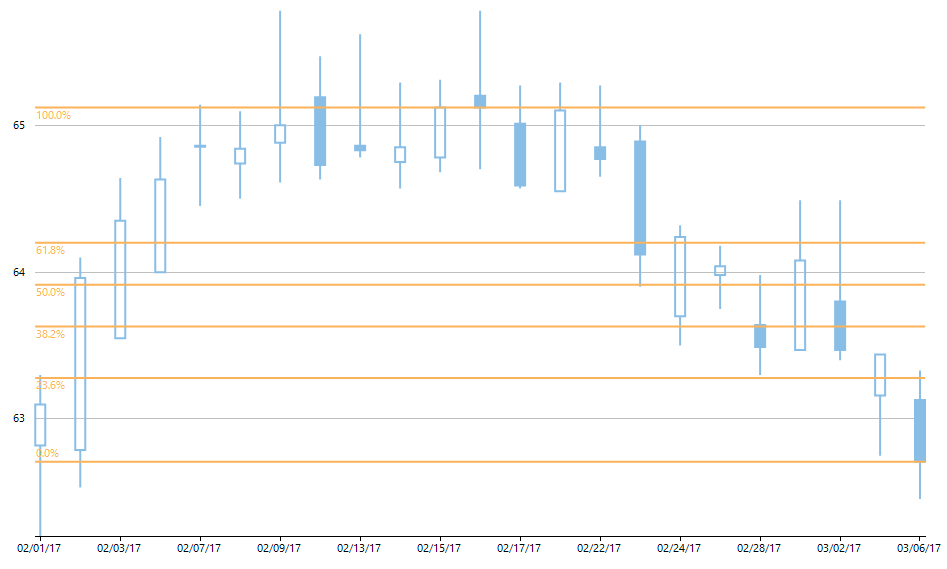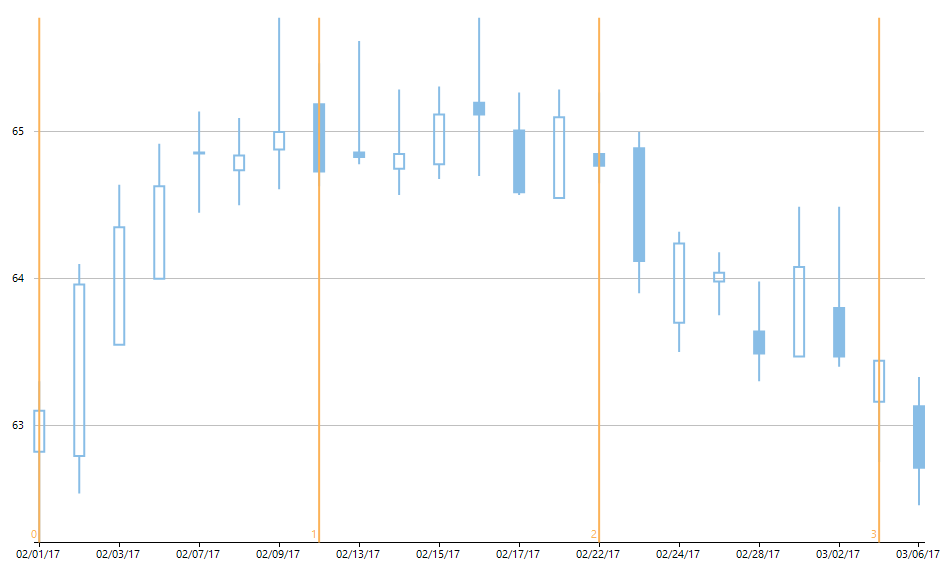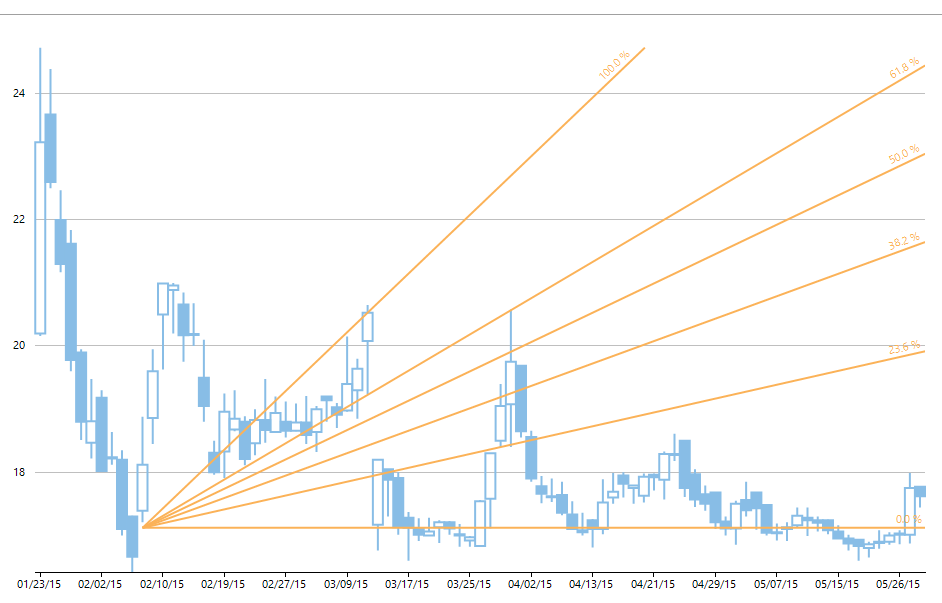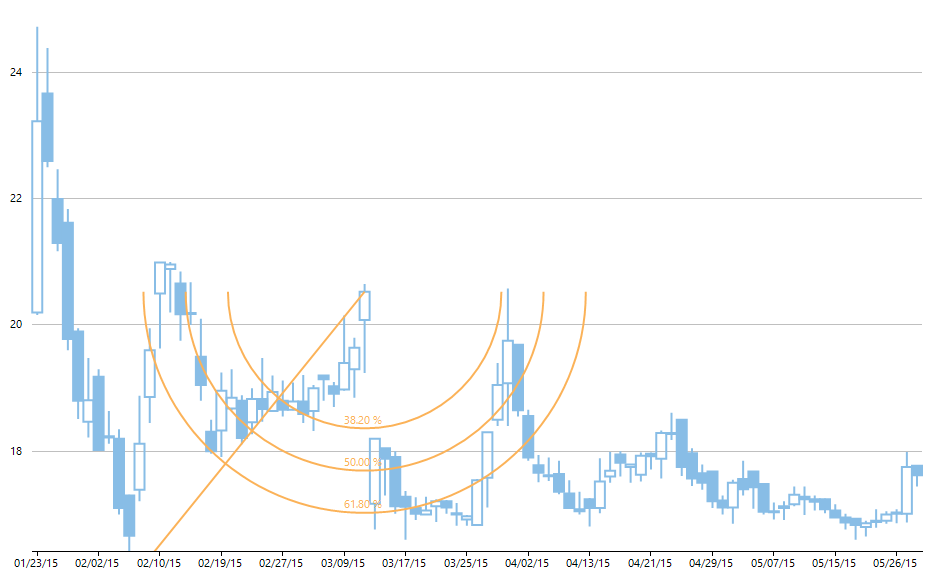ComponentOne Studio's FinancialChart control comes with built-in support for four types of Fibonacci tools that can be applied to a price or an indicator. These tools helps to analyze trends in the price chart and predict the next price. When the price goes up, they act as resistance levels, and vice-versa. In this blog, we'll look at how you can use different Fibonacci tools to enable the calculation of uptrends and downtrends and apply various alert levels in FinancialCharts.

## About the Fibonacci Series and the Golden Ratio

The Fibonacci series is a sequence of numbers: 0, 1, 1, 2, 3, 5, 8, 13, 21, 34, and so on, where the next number in the sequence is the sum of the previous two numbers. This number sequence was discovered by Leonardo Fibonacci, an Italian mathematician. As the sequence progresses, if a number is divided by the previous number, it produces a ratio. The ratio starts from 1.5, and as the number progresses. the relationship reaches the golden ratio (1.618) and thereafter, this ratio remains constant through the series values. When the price of a financial instrument trend upwards or downwards, the upward or downward impulse wave has a mathematical relationship to the one that preceded it. This mathematical relationship is based on the golden ratio of 1.618 calculated through a series of Fibonacci numbers. Fibonacci tools use this mathematical relationship to predict trend reversal.

## Fibonacci Tools in FinancialChart Control

### Fibonacci Retracements

Fibonacci retracements are created by taking two extreme points (usually high and low) on a stock chart and dividing the vertical distance by the key Fibonacci ratios of 23.6%, 38.2%, 50%, 61.8% and 100%. Once these levels are determined, horizontal lines are drawn and used to identify support and resistance levels. Retracement levels are used to alert traders of a potential trend reversal, resistance area or support area. After a significant price movement up or down, the new support and resistance levels are usually at or near these lines. The chart below visualizes Dell Technologies stock data with Fibonacci Retracements using ComponentOne Studio's FinancialChart.Fig 1.1: Fibonacci Retracements Fibonacci retracement levels are static prices that do not change, thus allowing quick and easy identification of support levels. As per the Fibonacci retracement theory, after the uptrend/downtrend, one can anticipate a correction in the stock to last up to the next/previous Fibonacci ratio. The most significant retracement level to watch is 61.8%, where the maximum selling happens for a limited time. This primarily happens within the same or the next session. Once this period of pullback zone is passed, new sellers starts entering the market causing an uptrend to continue after a brief pause. In the above chart, the stock price was at its lowest value of 62 on February 1,and it crossed the next retracement level (23.6%) in an uptrend, suggesting that the current trend will continue. Similar behavior is observed at 38.2%, 50% and 61.8% retracement levels and the price eventually rose to 65.8. At 100% retracement level, the stock prices were constant for eight sessions, fluctuating in the range of 65-66. Thereafter, the prices dropped from 65.4 to 62.5 in the next 10 sessions and all retracement levels (61.8%, 50%, 38.2% and 23.6%) were giving a clear signal to immediately sell the stock.

### Fibonacci Time Zones

Fibonacci Time Zones are indicators used by technical traders to identify the periods in which the price of an asset will experience a significant movement. They are a series of vertical lines spaced at Fibonacci intervals 0, 1, 2, 5, 8, 13, and so on. The underlying theory behind these Time Zones is that the trading signal of continuation or reversal of trend is expected to be on or near these vertical lines. Once a trader chooses a starting position on the chart, a vertical line is placed on every subsequent day that correspond to the position in the Fibonacci number sequence. The chart below visualizes Dell Technologies stock data with Fibonacci Time zones in FinancialChart.Fig 1.2: Fibonacci Time Zones Fibonacci Time Zone is the only indicator which is price independent in its calculation, while all other technical indicators analyze the price-action on time axis. The beginning of a new time zone (vertical line) is usually considered as an area for the beginning of a major market movement. A trader can also wait for the next-day candle, after the beginning of a new time zone, to confirm the continuation or reversal of the current trend. In the above chart, trader has chosen February 1 (stock was trading at 62.8) as the starting position for rendering Fibonacci Time Zones. The uptrend continued for the next few sessions, and the next time zone was rendered on February 10, with a clear signal of reversal of trend. From an uptrend, the stock price became constant in the range of 65, until the third time zone was rendered on February 22, with a clear signal of downtrend. Stock prices dropped from 65 to 63.2 in the next six sessions, and the fourth time zone was rendered on March 3, with no clear signal. The next day candle for March 6 gave a clear signal of the downtrend's continuation, indicating a strong possibility of further drop in stock prices.

### Fibonacci Fans

Fibonacci fans are three trend lines based on Fibonacci retracement levels that are used to measure the speed of a trend's movement (uptrend or downtrend). These are created by first drawing a trendline through two points (usually the high and low in a specified period), and then by dividing the vertical distance between these two points by the key Fibonacci ratios of 38.2%, 50% and 61.8%. Each of these divisions represents invisible points within the vertical distance which, when connected from the leftmost point, results in the creation of three fan lines. The chart below visualizes stock data rendering FinancialChart's Fibonacci fans.Fig 1.3: Fibonacci Fans The underlying theory behind these Fan lines is that the market will retrace a portion of the previous movement before continuing in the direction of the price trend and as the time lapses, the possibility of greater retracement diminishes. If price moves below a Fibonacci fan trendline, then price is usually expected to fall further until the next Fibonacci fan trendline. In the above chart, prices moved below 61.8% trendline and then moved below 50% as well. This gave a clear signal that the price will go further down and it did happen at 38.2% and 28.6% fan line as can be seen in the chart. Likewise, in a downtrend, if prices rise to a Fibonacci fan trendline, then that trendline is expected to act as a resistance. If that price is pierced then the next fan trendline is expected to act as resistance.

### Fibonacci Arcs

Fibonacci Arcs are three curved lines based on Fibonacci retracement points that are drawn for anticipating key support and resistance levels. These are created by first drawing an invisible trendline between two points (usually the high and low in a given period), and then by drawing three curves that intersect this trendline at the key Fibonacci ratios of 38.2%, 50% and 61.8%. The chart below visualizes stock data rendering FinancialChart's Fibonacci arcs.Fig 1.4: Fibonacci Arcs The underlying theory behind Fibonacci Arcs is that the transaction decisions (buying or selling) can be made when the price of a financial instrument crosses through these key levels. The price action in the above chart breaks the 38.20%, 50% and 61.8% Fibonacci arcs. After breakout in each arc, the stock price decreased further and the downtrend is expected to continue for next few sessions as well.

### Try the Fibonacci Tools Demo

Download Fibonacci Tools Sample: WinForms | WPF